# 四叉树优化碰撞检测

130 篇文章 0 订阅

## 四叉树原理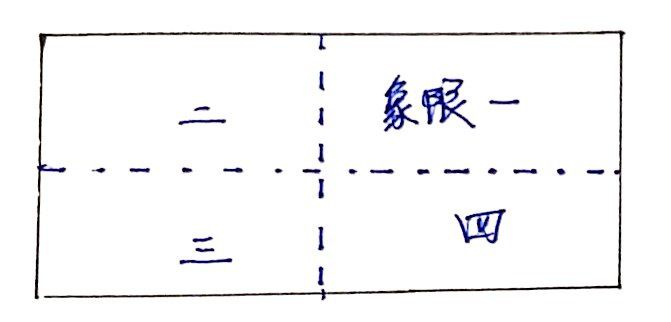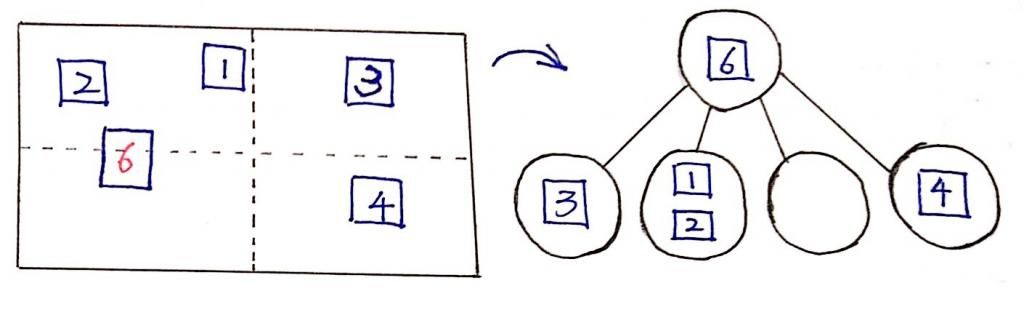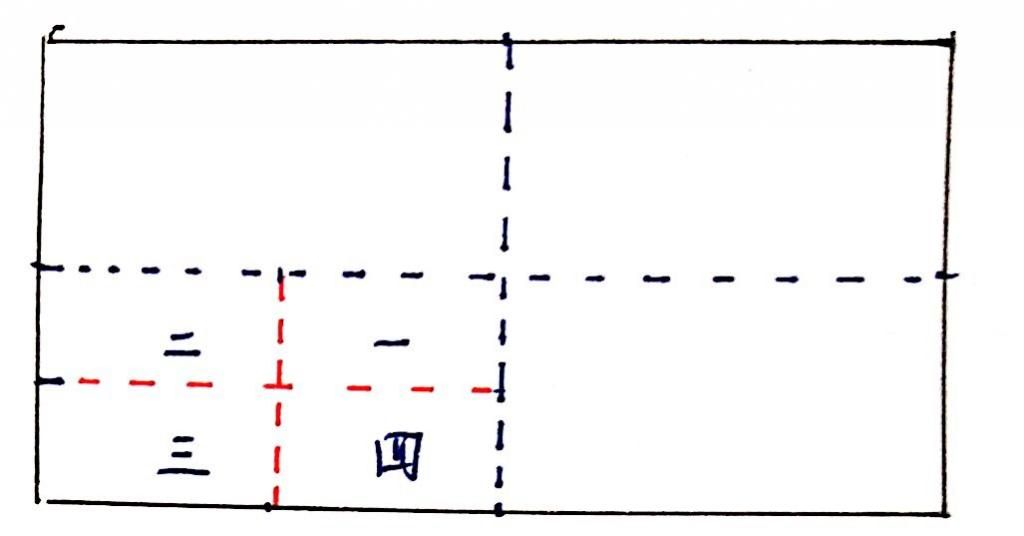## 实现四叉树

/*
四叉树节点包含：
- objects: 用于存储物体对象
- nodes: 存储四个子节点
- level: 该节点的深度，根节点的默认深度为0
- bounds: 该节点对应的象限在屏幕上的范围，bounds是一个矩形
*/
this.objects = [];
this.nodes = [];
this.level = typeof level === 'undefined' ? 0 : level;
this.bounds = bounds;
}

/*
常量：
- MAX_OBJECTS: 每个节点（象限）所能包含物体的最大数量
- MAX_LEVELS: 四叉树的最大深度
*/


/*
获取物体对应的象限序号，以屏幕中心为界限，切割屏幕:
- 右上：象限一
- 左上：象限二
- 左下：象限三
- 右下：象限四
*/
var bounds = this.bounds,
onTop = rect.y + rect.height <=  bounds.centroid.y,
onBottom = rect.y >= bounds.centroid.y,
onLeft = rect.x + rect.w <= bounds.centroid.x,
onRight = rect.x >= bounds.centroid.x;

if (onTop) {
if (onRight) {
return 0;
} else if (onLeft) {
return 1;
}
} else if (onBottom) {
if (onLeft) {
return 2;
} else if (onRight) {
return 3;
}
}

// 如果物体跨越多个象限，则放回-1
return -1;
};


// 划分
var level = this.level,
bounds = this.bounds,
x = bounds.x,
y = bounds.y,
sWidth = bounds.width / 2,
sHeight = bounds.height / 2;

this.nodes.push(
new QuadTree(new Rect(bounds.centroid.x, y, sWidth, sHeight), level + 1),
new QuadTree(new Rect(x, y, sWidth, sHeight), level + 1),
new QuadTree(new Rect(x, bounds.centroid.y, sWidth, sHeight), level + 1),
new QuadTree(new Rect(bounds.centroid.x, bounds.centroid.y, sWidth, sHeight), level + 1)
);
};


/*
插入功能：
- 如果当前节点[ 存在 ]子节点，则检查物体到底属于哪个子节点，如果能匹配到子节点，则将该物体插入到该子节点中
- 如果当前节点[ 不存在 ]子节点，将该物体存储在当前节点。随后，检查当前节点的存储数量，如果超过了最大存储数量，则对当前节点进行划分，划分完成后，将当前节点存储的物体重新分配到四个子节点中。
*/
var objs = this.objects,
i, index;

// 如果该节点下存在子节点
if (this.nodes.length) {
index = this.getIndex(rect);
if (index !== -1) {
this.nodes[index].insert(rect);
return;
}
}

// 否则存储在当前节点下
objs.push(rect);

// 如果当前节点存储的数量超过了MAX_OBJECTS
if (!this.nodes.length &&
this.objects.length > this.MAX_OBJECTS &&
this.level < this.MAX_LEVELS) {

this.split();

for (i = objs.length - 1; i >= 0; i--) {
index = this.getIndex(objs[i]);
if (index !== -1) {
this.nodes[index].insert(objs.splice(i, 1));
}
}
}
};


### 筛选功能

/*
检索功能：
给出一个物体对象，该函数负责将该物体可能发生碰撞的所有物体选取出来。该函数先查找物体所属的象限，该象限下的物体都是有可能发生碰撞的，然后再递归地查找子象限...
*/
var result = [],
index;

if (this.nodes.length) {
index = this.getIndex(rect);
if (index !== -1) {
resutl = result.concat(this.nodes[index].retrieve(rect));
}
}

result = result.concat(this.objects);

return result;
};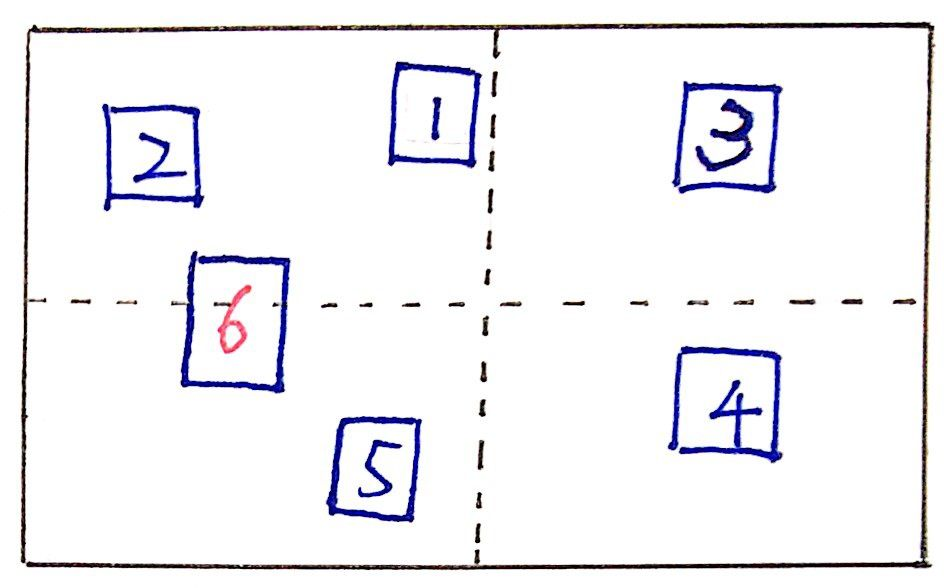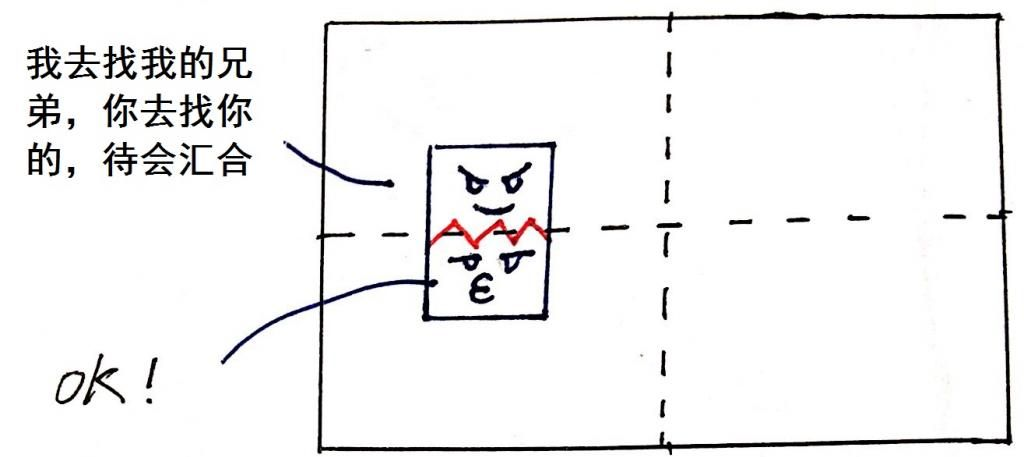// 检索
var result = [],
arr, i, index;

if (this.nodes.length) {
index = this.getIndex(rect);
if (index !== -1) {
resutl = result.concat(this.nodes[index].retrieve(rect));
} else {

// 切割矩形
arr = rect.carve(this.bounds);

for (i = arr.length - 1; i >= 0; i--) {
index = this.getIndex(arr[i]);
resutl = result.concat(this.nodes[index].retrieve(rect));

}
}
}

result = result.concat(this.objects);

return result;
}


### 动态更新

var tree = new Quadtree(new Rect(0, 0, 1000, 500));


var rectsArr = [/* ... */];
rectsArr.forEach(function(rect) {
tree.insert(rect);
});


rectsArr.forEach(function(rect) {
var result = tree.retrive(rect);
result.forEach(function() {
// norrow phase部分的碰撞检测...
});
})


var run = function run() {
// 重新向四叉树中插入所有物体，重新初始化四叉树
// ...

// 筛选物体集合并进行碰撞检测
// ...

requestAnimationFrame(run);
};

requestAnimationFrame(run);


// 判断矩形是否在象限范围内
function isInner(rect, bounds) {
return rect.x >= bounds.x &&
rect.x + width <= bounds.x + bounds.width &&
rect.y >= bounds.y &&
rect.y + rect.height <= bounds.y + bounds.height;
}

/*
动态更新：
从根节点深入四叉树，检查四叉树各个节点存储的物体是否依旧属于该节点（象限）的范围之内，如果不属于，则重新插入该物体。
*/
var objs = this.objects,
rect, index, i, len;

root = root || this;

for (i = objs.length - 1; i >= 0; i--) {
rect = objs[i];
index = this.getIndex(rect);

// 如果矩形不属于该象限，则将该矩形重新插入
if (!isInner(rect, this.bounds)) {
if (this !== root) {
root.insert(objs.splice(i, 1));
}

// 如果矩形属于该象限 且 该象限具有子象限，则
// 将该矩形安插到子象限中
} else if (this.nodes.length) {
this.nodes[index].insert(objs.splice(i, 1));
}
}

// 递归刷新子象限
for (i = 0, len = this.nodes.length; i < len; i++) {
this.nodes[i].refresh(root);
}
};


var run = function run() {
// 动态更新
tree.refresh();

// 筛选物体集合并进行碰撞检测
// ...

requestAnimationFrame(run);
};

requestAnimationFrame(run);


06-181151
07-12127711-15390
07-15375
06-0276
12-221万+
10-04538
03-24104
09-192758
03-294067
08-195390
06-091216
05-30364
07-302410
08-1199
04-17549点击重新获取扫码支付余额充值